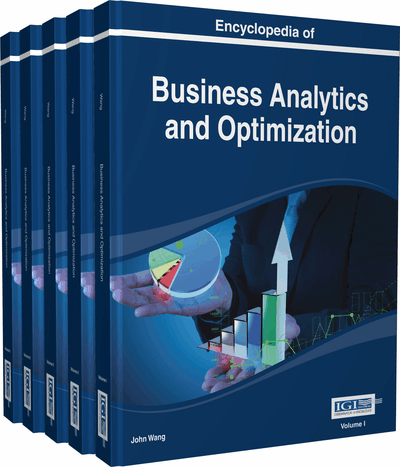# Nonlinear Programming

Xiaofeng Zhao (University of Mary Washington, USA)
DOI: 10.4018/978-1-4666-5202-6.ch147

Top

## Introduction

Nonlinear programming (NLP) deals with the problem of optimizing an objective function in the presence of equality and inequality constraints, where some of the constraints or the objective functions are nonlinear. A general optimization problem is to select n decision variablesfrom a given feasible region in such a way as to optimize (minimize or maximize) a given objective functionof the decision variables. The problem is called a nonlinear programming problem (NLP) if the objective function is nonlinear and/or the feasible region is determined by nonlinear constraints.

For notational convenience, we usually let x denote the vector of n decision variablesthat is, x = () and write the problem more concisely. Throughout the article, we shall consider the following NLP problem:Minimize f (x),Subject to

where X is a subset of, x is a vector ofcomponents, and f: XR, g: Xand h: Xare defined on X.

The function f is usually called the objective function. Each of the constraintsis called an inequality constraint, and each of the constraints, is called an equality constraint. Note also that the set X typically includes lower and upper bounds on the variables. The reason for separating variable bounds from the other inequality constraints is that they can play a useful role in some algorithms, i.e., they are handled in a specific way. A vectorsatisfying all the constraints is called a feasible solution to the problem; the collection of all such points forms the feasible region. The NLP problem, then, is to find a feasible pointsuch thatfor each feasible point x. Needless to say, a nonlinear programming problem can be stated as a maximization problem, and the inequality constraints can be written in the form.

## Key Terms in this Chapter

Infeasible Solution: A decision alternative or solution that does not satisfy one or more constraints.

Global Optimum: A feasible solution is a global optimal optimum if there are no other feasible points with better objective value in the entire feasible region. A global optimum may be either a global maximum or a global minimum.

Constraints: Restrictions or limitations imposed on a problem.

Convex Function: A function that is bowl-shaped up. For example, are convex functions.

Optimal Solution: The specific decision variable values or value that provide the best output for the model.

Concave Function: A function that is bowl-shaped down. For example, are convex functions.

Sensitivity analysis: The study of how changes in the coefficients of a nonlinear programming problem affect the optimal solution.

Local Optimization: A feasible solution is a local optimum if there are no other feasible solutions with a better function value in the immediate neighborhood. A local optimum may be either maximum or local minimum.

Objective Function: A mathematical expressions that describes the problem’s objective.

## Complete Chapter List

Search this Book:
Reset